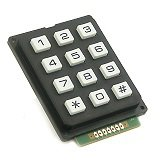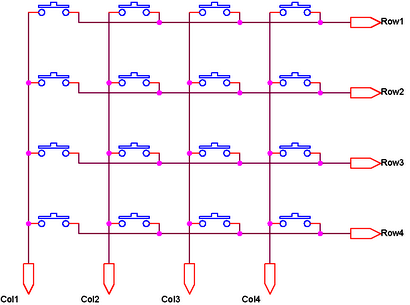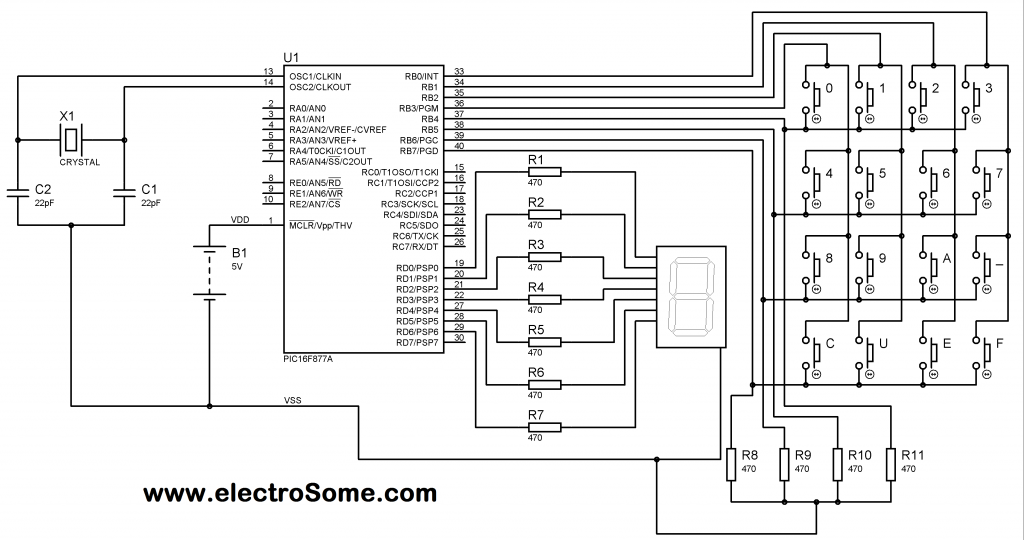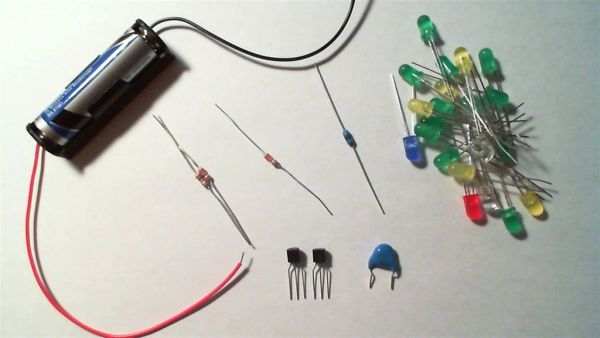# Interfacing Matrix Keypad with PIC Microcontroller

This tutorial explains ‘What is a Matrix Keypad ?’ and ‘How to Interface Matrix Keypad with PIC Microcontroller ?’.Matrix Keypad is a very useful and userfriendly when we want to design certain applications like Calculator, Telephone etc. Matrix Keypad is made by arranging push button switches in rows and columns. Just imagine, if you want to interface a 4*4 (16 keys) matrix keypad with a microcontroller.  In the straight forward way, you will need 16 pins of a microcontroller for that, but by using a simple technique we can reduce it to 8 pins. In the matrix keypad switches are connected in a special manner a shown in the figure below.Pressed keys can be detected by Scanning. For the sake of explanation, lets assume all column connections (Col1 – Col4) are input pins and all row connections (Row1 – Row4) are output pins. In the normal case (not scanning) all column inputs where in LOW (GND) state. For scanning keypad,

1. A Logic HIGH signal is given to Col1 of column inputs.
2. Then each Row output (row1 – row4) is scanned one by one. If any of the key belongs to first column is pressed, the Logic high signal from the Col1 will pass to that row. Through we can detect the key.
3. This process is repeated for all columns if we want to detect multiple keys.

In this post I am explaining only about detecting one key at a time. For explaining the working I am using a 4*4 matrix keypad and the result is displayed in a Seven Segment Display. Matrix Keypad scanning is stopped as soon as any key press is detected and the Scanning is restarted if we need more inputs.

READ  UART Communication using PIC Microcontroller

# Interfacing with PIC Microcontroller

Matrix Keypad can also be interfaced with PIC Microcontroller using MikroC Libraries.

## Circuit DiagramMatrix Keypad interfacing with PIC Microcontroller

Note: VDD and VSS of the pic microcontroller is not shown in the circuit diagram. VDD should be connected to +5V and VSS to GND.

Matrix Keypad is connected to the PORTB of the PIC Microcontroller. Each column of the Matrix Keypad is connected to RB0 – RB3 of the PIC Microcontroller, which are configured as output pins. While each row of the Matrix Keypad is connected to RB4 – RB7 of the PIC Microcontroller, which are configured as input pins.

For more detail: Interfacing Matrix Keypad with PIC Microcontroller